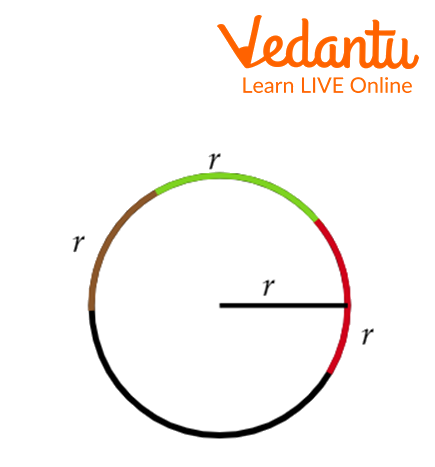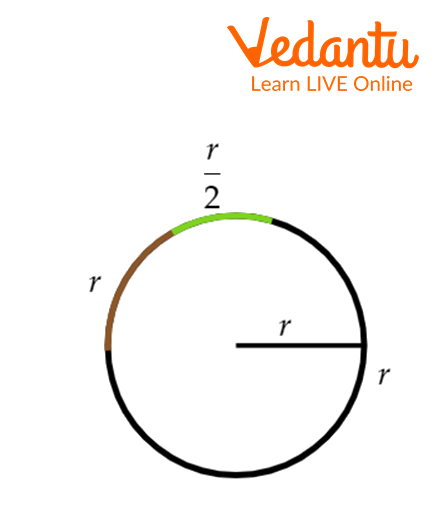Courses
Courses for Kids
Free study material
Free LIVE classes
More

# Relation between radian and real numbersLIVE
Join Vedantu’s FREE Mastercalss

## What is a radian?: An Introduction

We usually use the radian and degrees as units to measure an angle. The relation between radians and degrees is the relationship between radians and real numbers because the degree is a real number. Radian is used to measure the arc of a circle and is easy to calculate. The real number of a radian is not a whole number but is easy to visualize on the number line.

## The Radian of a Number System

Radian of a number system is known as the number of counting digits in a number system. For example, decimal number system has 10 digits, i.e., 0, 1, 2, …, 9. Thus the radian of decimal system is 10. Similarly Binary number system has a radian 2, octal has 8 and hexadecimal has 16.

## Relation between Radian and Real Numbers

The circumference of a circle is $2\pi r$, where r is the radius of the circle. The arc length of a circle with a radius of 1 unit is $2\pi$ radian. Again we know that $2\pi$ radian = 360.

Divide both sides by $2\pi$

$1\,radian = \dfrac{{360}}{{2\pi }}$

Putting the $\pi = \dfrac{{22}}{7}$

$1\,radian = \dfrac{{360}}{{2 \times \dfrac{{22}}{7}}}$

Solving the above equation:

$1\,radian = 57.296$

By solving the equation we get 1 radian = 57.296. The relationship between radian and real numbers is 1 radian = 57.296(approx.).

## What is the relationship between radians and real numbers?

1 radian of a circle with a radius r is equal to the central angle at the center whose arc is equal to r. The radians are at the same distance on the number line.

We know that the length of an arc of a circle is $\theta r$, where $\theta$ is in radians.

Again, the length of an arc of a circle is $\theta \times r \times \dfrac{{180}}{\pi }$ where $\theta$ is in degree.

 Radian Real Number 1 57.296 2 113.592 10 572.96 $\pi$ 180 $2\pi$ 360

## Interesting fact

• Radian is the S I unit used for measuring angles.

• The circumcircle of a circle is $2\pi$ radian.

## Solved examples

1. Visualize 3 radians on a circle.

Solution:

We know that one radian is equal to the central angle which makes an arc that length is equal to the radius of the circle.

Step 1: First we will draw a circle and measure the radius of the circle using a thread.

Step 2: Place the thread three times around the circle.2. Convert $3\pi$ in real number.

Solution: We know that 1 radian =57.296.

Thus multiply 57.296 with $3\pi$ to get it in real number.

$3\pi \times 57.296 = 171.88\pi$

Putting the value of $\pi$

$3\pi \times 57.296 = 171.88 \times \dfrac{{22}}{7}$

$3\pi \times 57.296 = 540.219$ (approx.)

3. Convert $75^{\circ}$ into radian.

Solution: Multiplying $\dfrac{\pi}{180^{\circ}}$ with $75^{\circ}$ to convert into radian:

$75^{\circ}=75^{\circ}\dfrac{\pi}{180^{\circ}}$

$\Rightarrow 75^{\circ}=\dfrac{5\pi}{12^{\circ}}$

Therefore $75^{\circ}=\dfrac{5\pi}{12^{\circ}}$

## Conclusion

Radian is the SI unit for measuring angles. Another unit for measuring angles is degrees. 1 radian = $\dfrac{180^{\circ}}{\pi}$ and $1^{\circ}=\dfrac{\pi}{180^{\circ}}$ radians. Radian measurements and real number measurements are the same.

The real value of one radian is 57.296(approx). The real value of radian is an irritation number.

## Practices problem:

1. Convert $4\pi$ radian into a real number.

2. Draw 1.5 radians on a circle.## List of related articles

Last updated date: 22nd Sep 2023
Total views: 38.1k
Views today: 1.38k

## FAQs on Relation between radian and real numbers

1. What is the relation between radius and arc length?

One radian is an angle that makes an arc equal length of the radius of the circle. We know that arc length = $r\theta$.

This implies, $\theta = \dfrac{{arc\,length}}{r}$

In case of 1 radian, $\theta = 1\,{\rm{radian}}$ and arc length = r

$1\,{\rm{radian}} = \dfrac{r}{r}$

$1\,{\rm{radian}} = 1$

Therefore, arc length = $r\theta$ , where $\theta$ is radian.

2. Why do we use radian?

Radians help to relate a linear measure and an angle measure. A unit circle is a circle that has a radius of 1 unit. The 1-unit radius is the same as 1 unit along the circumference.

3. How are radians used in real life?

Radians are more commonly used instead of degrees when measuring angles. In degrees, a complete revolution of a circle is 360⁰ however in radians it is $2\pi$. If an arc of the circle is drawn such that the radius is the same length of the arc, the angle created is 1 radian.